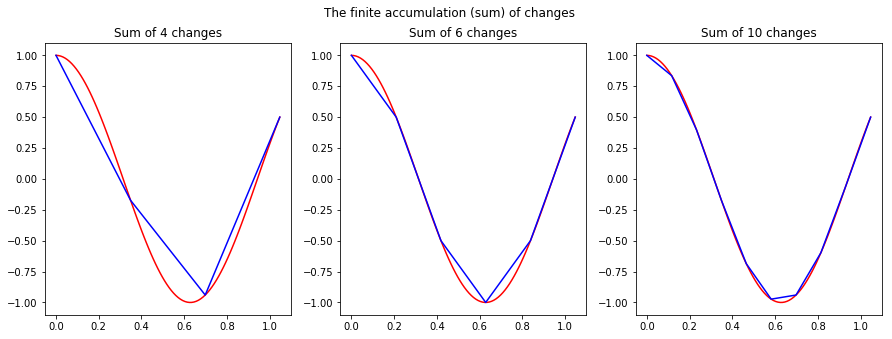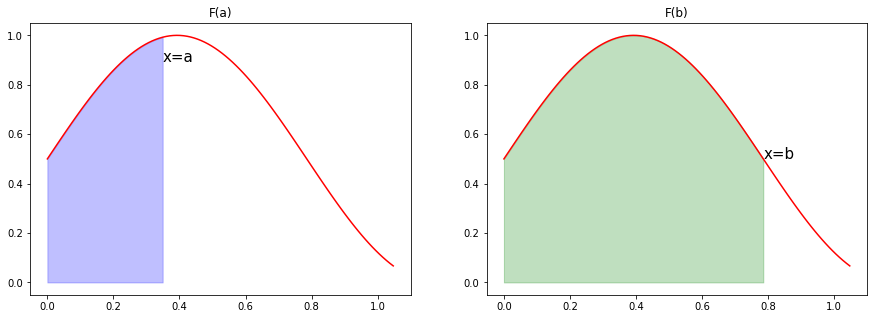# Fundamental Theorem of Calculus Explained

Relation between derivatives and integrals

# Why are derivatives the inverse of integrals?¶

#### By Sam Brunacini¶

Understandably, most people get confused when first introduced to the idea that finding the area under a curve is the inverse to finding instantaneous rate of change. It seems like the intuition behind this is hidden behind all the formulas they make you learn. Here I’ll explain why the Fundamental Theorem of Calculus (FTC) works. This is not a rigorous proof of the theorem, just an explanation of why they make sense.

First, let’s write the FTC: $$\text{Part 1: } \frac{d}{dx}\int_{a}^{x}f(t)dt = f(x)\\$$ $$\text{Part 2: } \int_{a}^{b}f(x)dx=F(b)-F(a)$$

To help understand Part 1, imagine you own a library. Before the library opens at 8:00 AM, you count 100 books in stock. Between 8:00 and 9:00, 5 books are withdrawn. Between 9:00 and 10:00, 8 books are returned. Without recounting, what is the number of books in stock at 10:00? Of course, you subtract 5 from 100 to account for the withdrawals then add 8 for the returns. This leaves $100 – 5 + 8 = 103$.

Believe it or not, this is exactly what FTC Part 1 says. Call the number of books in the library $x$ hours after 8:00 $f(x)$. Then $f(0)=100$ because that’s how many books there were before anyone came in. $f(x)$ changes by -5 during the first hour. We then add this change to the total to get $f(1)=95$. Due to the returns, $f(x)$ changes by 8 in the second hour. Adding this change to the total, we know $f(2)=103$. FTC part 1 says that a function is equal to the accumulation (or total) of the changes in itself as $x$ varies. The derivative indicates change, and the integral indicates accumulation, or summing the values together. This is exactly what happened in the library example.

In :
import matplotlib.pyplot as plt
import numpy as np
import math

THIRD_PI = math.pi / 3

plt.rcParams['figure.figsize'] = [15, 5]
fig, (ax1, ax2, ax3) = plt.subplots(1, 3)
fig.suptitle("The finite accumulation (sum) of changes")
ax1.set_title("Sum of 4 changes")
ax2.set_title("Sum of 6 changes")
ax3.set_title("Sum of 10 changes")

def plot_cos(axes, steps=100, color="blue"):
X = np.linspace(0, THIRD_PI, num=steps)
Y = np.cos(5*X)
axes.plot(X, Y, color=color)

plot_cos(ax1, color="red")
plot_cos(ax2, color="red")
plot_cos(ax3, color="red")

plot_cos(ax1, 4)
plot_cos(ax2, 6)
plot_cos(ax3, 10)

plt.show()The graphs above show finite sums of changes in some function. Roughly speaking, an integral is the sum of infinitely many tiny changes in the function. Notice that as the number of changes increases, the approximation (blue line) gets closer to the exact curve (red line). In fact, the approximation gets arbitrarily close to the curve as the number of changes grows larger.

Now, for Part 2. Remember that $F(x)$ is defined as the function that gives the area under a curve from 0 to $x$. Assume for now that $a \lt b$. This is a safe assumption since we can use the formula $$\int_{b}^{a}f(x)dx=-\int_{a}^{b}f(x)dx$$ to make it true if originally $a \gt b$. $F(a)$ is then the area under the curve from 0 to $a$ and $F(b)$ is the area under the curve from 0 to $b$. If we plot these separately, it looks like this:

In :
import matplotlib.pyplot as plt
import numpy as np
import math

THIRD_PI = math.pi / 3

plt.rcParams['figure.figsize'] = [15, 5]
fig, (ax1, ax2) = plt.subplots(1, 2)
ax1.set_title("F(a)")
ax2.set_title("F(b)")

def plot_sin(axes, steps=100, color="red"):
X = np.linspace(0, THIRD_PI, num=steps)
Y = np.sin(4*X)/2+0.5
axes.plot(X, Y, color=color)

plot_sin(ax1)
plot_sin(ax2)

X1 = np.linspace(0, THIRD_PI/3)
ax1.fill_between(X1, np.sin(4*X1)/2+0.5, color="blue", alpha=0.25)
ax1.annotate("x=a", xy=(THIRD_PI / 3, math.sin(4 * THIRD_PI / 3) / 2 + 0.4), size=15)

X2 = np.linspace(0, THIRD_PI*0.75)
ax2.fill_between(X2, np.sin(4*X2)/2+0.5, color="green", alpha=0.25)
ax2.annotate("x=b", xy=(THIRD_PI * 0.75, math.sin(3*THIRD_PI)/2+0.5), size=15)
plt.show()If you subtract the area shown in the first diagram from the second (which is simply doing $F(b) – F(a)$), you end up with the area between $a$ and $b$.

In [ ]: